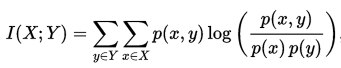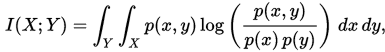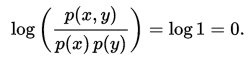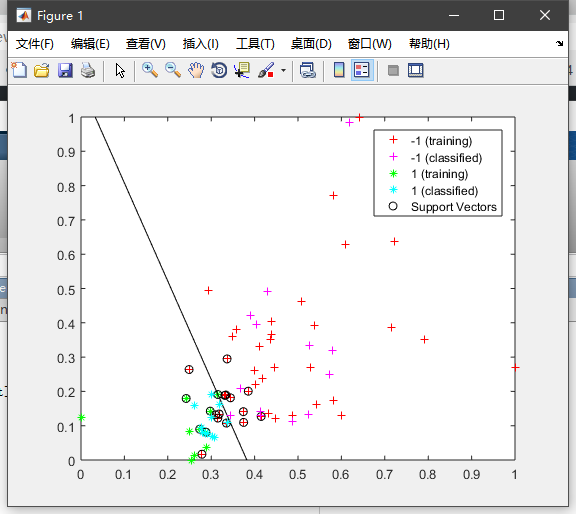• 2019-06-27 18:14:19

## 特征提取

### 1.TF-IDF学习

之前已经总结过TF-IDF相关的内容了。这里就不再重复编写了，需要的话请参看：https://blog.csdn.net/sir_TI/article/details/88135434。本质内容就是一个公式的问题。

然后现在主要总结的就是关于TF-IDF相关的代码分析。先总结出特征提取的流程，然后将相关的内容以代码的形式体现出来。

具体请参见我的github

### 2.互信息

说起互信息，其实我们都不陌生。相信当您学到了这儿的时候，已经对决策树有了基本的了解，那么现在就简单的回顾一下相关的概念：

#### 2.1 熵

如果X是一个离散型随机变量，取值空间为R，其概率分布为 p ( x ) = P ( X = x ) , x ⊆ R p(x)=P(X=x),x\subseteq R .那么X的熵的定义式为：
H ( X ) = − ∑ x ⊆ R p ( x ) log ⁡ 2 p ( x ) H(X)=-\sum _{x \subseteq R}p(x)\log_2p(x)
其中熵的物理意义就是反应了某个物体的混乱程度。当熵最大时，随机变量最不确定。

#### 2.2 联合熵

如果X,Y是一对离散型随机变量X,Y ~ P(x,y),X,Y的联合熵（joint entropy）定义为：
H ( X , Y ) = − ∑ x ⊂ X ∑ y ⊂ Y p ( x , y ) log ⁡ 2 p ( x , y ) H(X,Y)=-\sum _{x\subset X}\sum_{y\subset Y}p(x,y)\log_2p(x,y)
联合熵描述的意义就是一对随机随机变量平均所需要的信息量。

#### 2.3 条件熵

给定随机变量X的情况下，随机变量Y的条件熵（conditional entropy）定义为：
H ( Y ∣ X ) = − ∑ x ⊂ X ∑ y ⊂ Y p ( x , y ) log ⁡ 2 p ( y ∣ x ) H(Y|X)=-\sum _{x\subset X}\sum_{y\subset Y}p(x,y)\log_2p(y|x)

#### 2.4 互信息

在这里我先给出定义：如果随机变量(X,Y)~p(x,y),那么X，Y之间的互信息定义为：
I ( X ; Y ) = H ( X ) − H ( X ∣ Y ) I(X;Y)=H(X)-H(X|Y)
是不是很熟悉，其实我们所说的互信息，其实就是等同于决策树中定义的信息增益。

那么笔者上面讲了一堆内容，有什么用呢？还不如直接就是给出互信息的公式呢。那么请看我慢慢道来：

我们知道，条件概率的定义式为： P ( y ∣ x ) = p ( x , y ) p ( x ) P(y|x)=\frac{p(x,y)}{p(x)} ,那么我们对联合熵进行化简：
KaTeX parse error: No such environment: align at position 8: \begin{̲a̲l̲i̲g̲n̲}̲\nonumber H(X,Y…
同理可得：
H ( X , Y ) = H ( Y ) + H ( X ∣ Y ) H(X,Y)=H(Y)+H(X|Y)
因为联合熵是相等的，那么有：
H ( X ) + H ( Y ∣ X ) = H ( Y ) + H ( X ∣ Y ) H(X)+H(Y|X)=H(Y)+H(X|Y)
即:
H ( X ) − H ( X ∣ Y ) = H ( Y ) − H ( Y ∣ X ) H(X)-H(X|Y)=H(Y)-H(Y|X)
通过这样一系列的推导，我们就可以知道互信息（信息增益）是怎么来的。

#### 2.5 交叉熵

说到了上面的几个内容，那么不得不提及的就是交叉熵了。交叉熵在我们的机器学习、深度学习中广泛的被用做为代价函数，那么接下来主要就是介绍交叉熵的定义以及为什么代价函数会使用交叉熵损失函数。

##### 2.5.1 定义

如果一个随机变量X~p(x),存在q(x),使得q(x)为用于近似p(x)的概率分布，那么随机变量X和模型q之间的交叉熵(cross entropy)定义为：
H ( p , q ) = − ∑ x p ( x ) log ⁡ q ( x ) H(p,q)=-\sum _{x}p(x)\log q(x)
交叉熵的概念就是用来衡量模型与真实概率分布之间的差异的情况。在实际的应用中，模型的交叉熵越小，模型的表现越好。

##### 2.5.2 why cross entropy?

为什么需要交叉熵损失函数呢？这里主要是相对于MSE（均方误差损失函数）进行解释。

结论就是：由于梯度的原因，可以加快训练速度。

具体解释参看下面这个Blog:为什么使用交叉熵作为损失函数

### 3.使用互信息来作为特征筛选

回顾之前，对于中文的数据集处理的最多的就是去除停用词，基本没有对我们的数据来进行特征筛选。想想就觉得可怕。

PS：之前就在某国产最大的性价比手机厂商面试的问到了这个问题，空有一番理论，没得实践，正好借着这个机会，从互信息出发，来进行特征筛选。

特征，在机器学习领域是无比重要的，好的特征能够更加明确的表达出模型的特点。但是在实际情况中，找到好的模型一般是无比困难的，这其中涉及到数据清洗，数据分析以及特征选择，然后进行一大段的分析选择之后，才能得到我们需要的特征。

特征选择的方式主要分为三个类别：

#### 3.1 过滤法

过滤法只用于检验特征向量和目标（响应变量）的相关度，不需要任何的机器学习的算法，不依赖于任何模型，只是应用统计量做筛选：我们根据统计量的大小，设置合适的阈值，将低于阈值的特征剔除。

所以，我们的过滤法主要是一个数学过程。过滤法可以主要采用以下指标进行过滤：

方差分析、相关系数、假设检验（如卡方检验、F检验和t检验等）、互信息等

#### 3.2 包装法

包装法的解决思路是会选择一个目标函数来一步步的筛选特征。

最常用的包装法是递归消除特征法(recursive feature elimination)。递归消除特征法使用一个机器学习模型来进行多轮训练，每轮训练后，消除若干权值系数的对应的特征，再基于新的特征集进行下一轮训练。在sklearn中存在RFE这个类来进行特征筛选。

#### 3.3 嵌入法

​ 嵌入法也是用机器学习的方法来选择特征，但是它和RFE的区别是它不是通过不停的筛掉特征来进行训练，而是使用的都是特征全集。在sklearn中，使用SelectFromModel函数来选择特征。

最常用的是使用L1正则化和L2正则化来选择特征。此外，GBDT和决策树也可以来选择特征。

另外一个常见的就是做的特征融合。就是根据特征之间的关系进行特征融合，找到更能表达出模型的特征。

### 3.4 实例

这部分主要以代码的形式展现，参见我的githubtf-idf
更多相关内容
• 联合互信息特征选择的python实现，这是参考文献（Feature selection based on joint mutual information）
• 综述性论文，如何使用互信息来进行特征选择。特征工程是机器学习最重要的领域之一，本文深入浅出介绍了特征选择的一个方向。
• 基于并行化互信息特征选择模块。 相关博客文章 依赖关系 scipy(>=0.17.0) numpy(>=1.10.4) scikit-learn(>=0.17.1) 瓶颈（>=1.1.0） 如何使用 像使用任何其他 scikit-learn 方法一样下载、导入和执行以下操作...Python
• 提出了一种基于条件互信息的图像特征选择方法。为了预测条件互信息,该方法选择与已选定特征具有最大熵的那些特征,并将选择出的特征进一步用于数字图像识别。图像识别器由支持向量机实现。实验中,识别器的输入数据是...
• 主成分分析是一种常用的特征选择算法, 经典方法是计算各个特征之间的相关, 但是相关无法评估变量间 的非线性关系. 互信息可用于衡量两个变量间相互依赖的强弱程度, 且不局限于线性相关, 鉴于此, 提出一种基于互...
• 论文研究一种基于类差分度的互信息特征选择方法.zip
• 在概率论和信息论中，两个随机变量的互信息(Mutual Information，简称MI)或转移信息(transinformation)是变量间相互依赖性的量度。不同于相关系数，互信息并不局限于实值随机变量，它更加一般且决定着联合分布 p(X,Y...

在概率论和信息论中，两个随机变量的互信息(Mutual Information，简称MI)或转移信息(transinformation)是变量间相互依赖性的量度。不同于相关系数，互信息并不局限于实值随机变量，它更加一般且决定着联合分布 p(X,Y) 和分解的边缘分布的乘积 p(X)p(Y) 的相似程度。互信息(Mutual Information)是度量两个事件集合之间的相关性(mutual dependence)。互信息最常用的单位是bit。

互信息的定义

正式地，两个离散随机变量 X 和 Y 的互信息可以定义为：

其中 p(x,y) 是 X 和 Y 的联合概率分布函数，而p(x)和p(y)分别是 X 和 Y 的边缘概率分布函数。在连续随机变量的情形下，求和被替换成了二重定积分：其中 p(x,y) 当前是 X 和 Y 的联合概率密度函数，而p(x)和p(y)分别是 X 和 Y 的边缘概率密度函数。

互信息量I(xi;yj)在联合概率空间P(XY)中的统计平均值。 平均互信息I(X;Y)克服了互信息量I(xi;yj)的随机性,成为一个确定的量。如果对数以 2 为基底，互信息的单位是bit。

直观上，互信息度量 X 和 Y 共享的信息：它度量知道这两个变量其中一个，对另一个不确定度减少的程度。例如，如果 X 和 Y 相互独立，则知道 X 不对 Y 提供任何信息，反之亦然，所以它们的互信息为零。在另一个极端，如果 X 是 Y 的一个确定性函数，且 Y 也是 X 的一个确定性函数，那么传递的所有信息被 X 和 Y 共享：知道 X 决定 Y 的值，反之亦然。因此，在此情形互信息与 Y(或 X)单独包含的不确定度相同，称作 Y(或 X)的熵。而且，这个互信息与 X 的熵和 Y 的熵相同。(这种情形的一个非常特殊的情况是当 X 和 Y 为相同随机变量时。)

互信息是 X 和 Y 联合分布相对于假定 X 和 Y 独立情况下的联合分布之间的内在依赖性。于是互信息以下面方式度量依赖性：I(X; Y) = 0 当且仅当 X 和 Y 为独立随机变量。从一个方向很容易看出：当 X 和 Y 独立时，p(x,y) = p(x) p(y)，因此：此外，互信息是非负的(即 I(X;Y) ≥ 0; 见下文)，而且是对称的(即 I(X;Y) = I(Y;X))。

互信息特征选择算法的步骤①划分数据集

②利用互信息对特征进行排序

③选择前n个特征利用SVM进行训练

④在测试集上评价特征子集计算错误率

缺点

此种特征选择方法是最大化特征与分类变量之间的相关度，就是选择与分类变量拥有最高相关度的前k个变量。但是，在特征选择中，单个好的特征的组合并不能增加分类器的性能，因为有可能特征之间是高度相关的，这就导致了特征变量的冗余。

代码

注意使用的数据集是dlbcl，大概五千多维，可以从UCI上下载，最终选择前100特征进行训练。

主函数代码：

MATLAB

1

2

3

4

5

6

7

8

9

10

11

12

13

14

15

16

17

18

19clear all

close all

clc;

[X_train,Y_train,X_test,Y_test] = divide_dlbcl();

Y_train(Y_train==0)=-1;

Y_test(Y_test==0)=-1;

% number of features

numF = size(X_train,2);

[ ranking , w] = mutInfFS( X_train, Y_train, numF );

k = 100; % select the Top 2 features

svmStruct = svmtrain(X_train(:,ranking(1:k)),Y_train,'showplot',true);

C = svmclassify(svmStruct,X_test(:,ranking(1:k)),'showplot',true);

err_rate = sum(Y_test~= C)/size(X_test,1); % mis-classification rate

conMat = confusionmat(Y_test,C); % the confusion matrix

fprintf('\nAccuracy: %.2f%%, Error-Rate: %.2f \n',100*(1-err_rate),err_rate);

mutInfFS.m

MATLAB

1

2

3

4

5

6

7

8

9

10function [ rank , w] = mutInfFS( X,Y,numF )

rank = [];

for i = 1:size(X,2)

rank = [rank; -muteinf(X(:,i),Y) i];

end;

rank = sortrows(rank,1);

w = rank(1:numF, 1);

rank = rank(1:numF, 2);

end

muteinf.m

MATLAB

1

2

3

4

5

6

7

8

9

10

11

12

13

14

15

16

17

18

19

20

21

22

23

24

25

26

27

28

29

30

31

32

33

34

35

36

37

38

39

40

41

42

43

44

45

46

47

48

49

50

51

52

53

54

55

56

57

58function info = muteinf(A, Y)

n = size(A,1);%实例数量

Z = [A Y];%所有实例的维度值及标签

if(n/10 > 20)

nbins = 20;

else

nbins = max(floor(n/10),10);%设置区间的个数

end;

pA = hist(A, nbins);%min(A)到max(A)划分出nbins个区间出来，求每个区间的概率

pA = pA ./ n;%除以实例数量

i = find(pA == 0);

pA(i) = 0.00001;%不能使某一区间的概率为0

od = size(Y,2);%一个维度

cl = od;

%下面是求实例不同标签的的概率值，也就是频率

if(od == 1)

pY = [length(find(Y==+1)) length(find(Y==-1))] / n;

cl = 2;

else

pY = zeros(1,od);

for i=1:od

pY(i) = length(find(Y==+1));

end;

pY = pY / n;

end;

p = zeros(cl,nbins);

rx = abs(max(A) - min(A)) / nbins;%每个区间长度

for i = 1:cl

xl = min(A);%变量的下界

for j = 1:nbins

if(i == 2) && (od == 1)

interval = (xl <= Z(:,1)) & (Z(:,2) == -1);

else

interval = (xl <= Z(:,1)) & (Z(:,i+1) == +1);

end;

if(j < nbins)

interval = interval & (Z(:,1) < xl + rx);

end;

%find(interval)

p(i,j) = length(find(interval));

if p(i,j) == 0 % hack!

p(i,j) = 0.00001;

end

xl = xl + rx;

end;

end;

HA = -sum(pA .* log(pA));%计算当前维度的信息熵

HY = -sum(pY .* log(pY));%计算标签的信息熵

pA = repmat(pA,cl,1);

pY = repmat(pY',1,nbins);

p = p ./ n;

info = sum(sum(p .* log(p ./ (pA .* pY))));

info = 2 * info ./ (HA + HY);%计算互信息

前100个特征的效果：

Accuracy: 86.36%, Error-Rate: 0.14

选择前两个特征进行训练(压缩率接近100%,把上述代码中的K设为2即可)的二维图：Accuracy: 75.00%, Error-Rate: 0.25

网站所有原创代码采用Apache 2.0授权

网站文章采用知识共享许可协议BY-NC-SA4.0授权

© 2018 · OmegaXYZ–版权所有 转载请注明出处 -皖ICP备 17007601

展开全文• 一种基于类差分度的互信息特征选择方法，任军，葛卫丽，通过引入类差分度，提出一种改进的互信息特征选择方法，并同时引入相对词频因子解决传统方法倾向于选择低频词的不足，合理地改善
• 我们现在处于一个数据驱动的时代，但并不是所有的...基于熵的特征选择方法就是选择一个能提供给类别尽可能多"信息"的特征子集，从而得到关于类别更多的"信息"，进而为分类提供帮助。如何衡量数据所携带信息的多少...

我们现在处于一个数据驱动的时代，但并不是所有的数据都是有意义的。只有有效的数据才能

带给我们"信息"，无效的数据并不能带给我们"信息"。

如果我们在做一件事情之前，可以通过各种数据得到这件事情所有相关信息，那么我们就可以

最初最完美的决策，使利益最大化。

基于熵的特征选择方法就是选择一个能提供给类别尽可能多"信息"的特征子集，从而得到关于类别更多的"信息"，进而为分类提供帮助。

如何衡量数据所携带信息的多少呢，克劳德·艾尔伍德·香农给出了熵的概念，通过熵我们可以

衡量数据携带信息的多少。香农

熵的定义如下：举一个简单的例子：如果有人告诉你11点过60分钟是12点，你一定觉得他说的是废话；但是

如果有人告诉你你的期末成绩优秀，你一定很感兴趣。这是因为前者的概率因此它的熵为0，所以给你提供的信息也是0。

而后者的概率(不及格，及格，中等，良好，优秀)

因此这件事情的熵为0.69，所以会提供给你一些信息。熵

第一步先介绍一下熵的相关概念。维恩图

上图,表示事件,的信息；被称为条件熵：表示在知道事件的情况下，此时再知道事件可以提供给我们

的信息。被称为互信息：表示事件共同提供的信息；也可以理解为知道了事件可以对事件提供多少信息，反之亦然。被称为联合熵：表示知道事件之后可以提供给我们的信息。

其中有：(怎么计算等公式我就不写了，主要是理解各种信息的关系)特征选择

这部分稍微谈一下特征选择方法。特征选择方法根据是否与后续的分类器交互分为过滤式、嵌

入式以及包裹式。其中过滤式特征选择不需要与分类器交互，而其他两种都需要和分类器进行

交互。特征选择流程

特征选择主要分为子集生成、子集评价、停止准则三大部分。

子集生成主要是如何选择特征，例如穷举法，前向选择、后向消除、启发式选择等等。

子集评价中有很多度量方法：准确率、距离、相关性、互信息等等；使用不同的度量方法最终

得到的特征子集也不一样。

停止准则是满足什么条件的时候停止特征选择过程，通常有特征子集达到一定的大小，选择阈

值以上的特征等等。基于互信息的特征选择方法

OK，当你对上面两部分有了了解之后，这部分就来说一下基于互信息的特征选择方法。

基于互信息的特征选择方法选择特征的过程比较简单。子集生成使用前向生成法，子集评价这

部分等下要讨论的部分，停止准则一般为特征子集大小到达一个预设值就停止。举个例子说：

我们数据集有100个特征，我们要选择20个，那么此方法就会遍历特征20次，每次都根据评价

准则选择最优的特征放入特征子集。

假设我们数据集特征为，类别为。如何设置评价标准才比较合理。

最简单的想法就是只考虑每个特征和类别之间的互信息，根据互信息的大小对特征

排序，然后选择最前面的个特征作为特征子集。这种特征选择方法也被称为MIM。其中为候选的特征。但是MIM方法有很明显的缺点，只考虑了特征和类别之间的相关性，没有考虑类别和类别的相

关性，机会造成特征子集的冗余；也就是可能出现值都很大，但是也很大(甚至接近1)，这种情况取其一即可。为了解决冗余性的问题，又

有两种不同的算法MIFS和MRMR被提出:其中为已经选择的特征，表示的大小。这样在选择新特征的时候不仅考虑了候选

特征和类别之间的互信息，也考虑了候选特征和已选特征之间的相关性，减少了冗余度。事情

到这里似乎已经很完美了，毕竟及考虑了相关性，也考虑了冗余性。然而，还有一个问题需要

考虑。

我们选择特征子集的首要目标是希望特征子集针对类别的相关性高，冗余性小。但是是两个特征之间的互信息，并没有考虑到类别。进一步说，两个特征之间的冗余

大，并不一定能说明这两个特征在类别上的冗余也大。据此，一系列考虑类内冗余的方法

也被提出，例如CIFE算法：第二项为类内特征冗余，即我们知道了类别信息，不同特征再次基础上的互信息。

下面再看一个维恩图：其中表示类别的信息量，表示已选择特征子集表示候选特征。

最终的目标就是希望可以用尽可能的少的特征，使得较大。上图中：但是其中这部分和类别没有关系，也就说这部分信息对于类别是无用的，我们

需要的只有类内冗余这部分，也就是其他关于降维的文章：超爱学习：10种常用降维算法源代码(python)​zhuanlan.zhihu.com超爱学习：你知道PCA的四种写法么​zhuanlan.zhihu.com展开全文• 基于模糊粗糙集的信息熵模型提出最大互信息最大相关熵标准，并根据该标准设计了一种新的特征选择方法，能同时处理离散数据、连续数据和模糊数据等混合信息。经UCI数据集试验，表明该算法与其他算法相比，具有较高的...
• 在概率论和信息论中，两个随机变量的互信息（Mutual Information，简称MI）或转移信息（transinformation）是变量间相互依赖性的量度。不同于相关系数，互信息并不局限于实值随机变量，它更加一般且决定着联合分布 p...

在概率论和信息论中，两个随机变量的互信息（Mutual Information，简称MI）或转移信息（transinformation）是变量间相互依赖性的量度。不同于相关系数，互信息并不局限于实值随机变量，它更加一般且决定着联合分布 p(X,Y) 和分解的边缘分布的乘积 p(X)p(Y) 的相似程度。互信息(Mutual Information)是度量两个事件集合之间的相关性(mutual dependence)。互信息最常用的单位是bit。

互信息的定义
正式地，两个离散随机变量 X 和 Y 的互信息可以定义为：

其中 p(x,y) 是 X 和 Y 的联合概率分布函数，而p(x)和p(y)分别是 X 和 Y 的边缘概率分布函数。在连续随机变量的情形下，求和被替换成了二重定积分：其中 p(x,y) 当前是 X 和 Y 的联合概率密度函数，而p(x)和p(y)分别是 X 和 Y 的边缘概率密度函数。

互信息量I(xi;yj)在联合概率空间P(XY)中的统计平均值。 平均互信息I(X;Y)克服了互信息量I(xi;yj)的随机性,成为一个确定的量。如果对数以 2 为基底，互信息的单位是bit。

直观上，互信息度量 X 和 Y 共享的信息：它度量知道这两个变量其中一个，对另一个不确定度减少的程度。例如，如果 X 和 Y 相互独立，则知道 X 不对 Y 提供任何信息，反之亦然，所以它们的互信息为零。在另一个极端，如果 X 是 Y 的一个确定性函数，且 Y 也是 X 的一个确定性函数，那么传递的所有信息被 X 和 Y 共享：知道 X 决定 Y 的值，反之亦然。因此，在此情形互信息与 Y（或 X）单独包含的不确定度相同，称作 Y（或 X）的熵。而且，这个互信息与 X 的熵和 Y 的熵相同。（这种情形的一个非常特殊的情况是当 X 和 Y 为相同随机变量时。）

互信息是 X 和 Y 联合分布相对于假定 X 和 Y 独立情况下的联合分布之间的内在依赖性。于是互信息以下面方式度量依赖性：I(X; Y) = 0 当且仅当 X 和 Y 为独立随机变量。从一个方向很容易看出：当 X 和 Y 独立时，p(x,y) = p(x) p(y)，因此：此外，互信息是非负的（即 I(X;Y) ≥ 0; 见下文），而且是对称的（即 I(X;Y) = I(Y;X)）。

更多互信息内容请访问：http://www.omegaxyz.com/2018/08/02/mi/

互信息特征选择算法的步骤
①划分数据集
②利用互信息对特征进行排序
③选择前n个特征利用SVM进行训练
④在测试集上评价特征子集计算错误率
代码
注意使用的数据集是dlbcl，大概五千多维，可以从UCI上下载，最终选择前100特征进行训练。

主函数代码：

clear all
close all
clc;
[X_train,Y_train,X_test,Y_test] = divide_dlbcl();
Y_train(Y_train==0)=-1;
Y_test(Y_test==0)=-1;
% number of features
numF = size(X_train,2);

[ ranking , w] = mutInfFS( X_train, Y_train, numF );
k = 100; % select the Top 2 features
svmStruct = svmtrain(X_train(:,ranking(1:k)),Y_train,'showplot',true);
C = svmclassify(svmStruct,X_test(:,ranking(1:k)),'showplot',true);
err_rate = sum(Y_test~= C)/size(X_test,1); % mis-classification rate
conMat = confusionmat(Y_test,C); % the confusion matrix
fprintf('\nAccuracy: %.2f%%, Error-Rate: %.2f \n',100*(1-err_rate),err_rate);

mutInfFS.m

function [ rank , w] = mutInfFS( X,Y,numF )
rank = [];
for i = 1:size(X,2)
rank = [rank; -muteinf(X(:,i),Y) i];
end;
rank = sortrows(rank,1);
w = rank(1:numF, 1);
rank = rank(1:numF, 2);

end

muteinf.m

function info = muteinf(A, Y)
n = size(A,1);%实例数量
Z = [A Y];%所有实例的维度值及标签
if(n/10 > 20)
nbins = 20;
else
nbins = max(floor(n/10),10);%设置区间的个数
end;
pA = hist(A, nbins);%min(A)到max(A)划分出nbins个区间出来，求每个区间的概率
pA = pA ./ n;%除以实例数量

i = find(pA == 0);
pA(i) = 0.00001;%不能使某一区间的概率为0

od = size(Y,2);%一个维度
cl = od;
%下面是求实例不同标签的的概率值，也就是频率
if(od == 1)
pY = [length(find(Y==+1)) length(find(Y==-1))] / n;
cl = 2;
else
pY = zeros(1,od);
for i=1:od
pY(i) = length(find(Y==+1));
end;
pY = pY / n;
end;
p = zeros(cl,nbins);
rx = abs(max(A) - min(A)) / nbins;%每个区间长度
for i = 1:cl
xl = min(A);%变量的下界
for j = 1:nbins
if(i == 2) && (od == 1)
interval = (xl <= Z(:,1)) & (Z(:,2) == -1);
else
interval = (xl <= Z(:,1)) & (Z(:,i+1) == +1);
end;
if(j < nbins)
interval = interval & (Z(:,1) < xl + rx);
end;
%find(interval)
p(i,j) = length(find(interval));

if p(i,j) == 0 % hack!
p(i,j) = 0.00001;
end

xl = xl + rx;
end;
end;
HA = -sum(pA .* log(pA));%计算当前维度的信息熵
HY = -sum(pY .* log(pY));%计算标签的信息熵
pA = repmat(pA,cl,1);
pY = repmat(pY',1,nbins);
p = p ./ n;
info = sum(sum(p .* log(p ./ (pA .* pY))));
info = 2 * info ./ (HA + HY);%计算互信息

前100个特征的效果：

Accuracy: 86.36%, Error-Rate: 0.14

选择前两个特征进行训练（压缩率接近100%,把上述代码中的K设为2即可）的二维图：Accuracy: 75.00%, Error-Rate: 0.25

更多内容访问omegaxyz.com
网站所有代码采用Apache 2.0授权
网站文章采用知识共享许可协议BY-NC-SA4.0授权

展开全文机器学习 MATLAB
• 特征选择主要从两个方面入手：特征是否发散：特征发散说明特征的方差大，能够根据取值的差异化度量目标信息.特征与目标相关性：优先选取与目标高度相关性的.对于特征选择，有时候我们需要考虑分类变量和连续变量的...
• 为了实现对模式识别、信号处理等领域中数据的有效表达，提出了一种基于规范互信息和动态冗余信号识别技术的特征选择方法。该方法采用规范互信息对特征相关性和冗余性进行测量，并通过一种动态冗余信号识别技术在特征...
• 因此，提出新的基于前向搜索的非线性特征选择算法，该算法使用互信息和交互信息的理论，寻找与多分类标签相关的最优子集，并降低计算复杂度。在UCI中9个数据集和4个不同的分类器对比实验中表明，该算法均优于原始...特征交互 特征冗余
• 文章目录1 基本概念信息量信息熵条件熵互信息量2 Python实现-特征选择过滤器mutual_info_classif：离散目标变量的互信息mutual_info_regression：连续目标变量的互信息 衡量单变量的相关性指标有很多，比如Pearson...python 机器学习
• 特征选择（卡方检验与互信息特征选择的主要目的有两点： 减少特征数量提高训练速度，这点对于一些复杂模型来说尤其重要 减少noisefeature以提高模型在测试集上的准确性。一些噪音特征会导致模型出现错误的泛化...人工智能 机器学习
• 为了在特征选择过程中得到较优的特征子集, 结合标准化互信息和遗传算法提出了一种新的两阶段特征选择方法。该方法首先采用标准化的互信息对特征进行排序, 然后用排序在前的特征初始化第二阶段遗传算法的部分种群, ...
• 基于模糊粗糙集的信息熵模型提出最大互信息最大相关熵标准，并根据该标准设计了一种新的特征选择方法，能同时处理离散数据、连续数据和模糊数据等混合信息。经UCI数据集试验，表明该算法与其他算法相比，具有较高的...模糊粗糙集 信息熵 相关熵
• 本文介绍的互信息（mutual information）即为过滤式的特征选择算法。 关于过滤式的特征算法系列，可参考我的其他文章。 特征选择之卡方检验 特征选择之Fisher Score 2、互信息 互信息介绍 定义：两个随机变量的...
• 这里介绍两种常用的特征选择方法： 互信息 　一个常用的方法是计算文档中的词项t与文档类别c的互信息MI，MI度量的是词的存在与否给类别c带来的信息量，互信息的基本定义如下： 　应用到文本特征选择:自然语言处理 nlp
• 描述： 用于以下基于MI的特征选择方法的代码（Matlab / C ++ Mex）： - 最大相关性 (maxRel) - 最小冗余最大相关性（MRMR） - 最小冗余 (minRed) - 二次编程特征选择 (QPFS) - 互信息商（MIQ） - 最大相关最小总冗余...matlab
• 研究了下sklearn.feature_selection()中参考的Estimating Mutual Information论文与Mutual Information between Discrete and Continuous Data Sets论文，整理一篇基于k-最近邻的互信息算法。k最近邻
• 本文引自西北工业大学杨宏晖老师的《模式识别之特征选择》 1、信息增益准则 对一个特征而言，系统有它和没有它时信息量将发生变化，而前后信息量的差值就是这个特征给系统带来的信息量，即增益。衡量标准是看特征...信息增益
• 一种互信息文本特征选择算法的改进，彭君睿，徐蔚然，文本分类问题中，特征选择是其中很重要的一个环节，互信息方法用于文本特征选择中的效果相比其他文本特征选择方法并不是很好，主文本分类
• 重新构造能实现分类属性数据信息量、条件互信息、特征之间依赖度定义的计算公式，并在此基础上，提出了一种基于互信息较大相关、较小冗余的特征选择（MRLR）算法。MRLR算法在特征选择时不仅考虑了特征与类标签之间的......